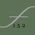## Friday, September 18, 2009

I was working on a date/time format in Flash and needed a way to pad values out so they always took up the same amount of space. For example making sure a month always has two digits. Making sure it displays a "05" instead of "5". I couldn't find anything built into AS2 or AS3 to do this so I wrote something that would. It allows you to pass in a value and pad either the left or right side up to a given amount of characters.

```/**
* This function will pad the left or right side of any variable passed in
* elem [AS object]
* finalLength: Number
* dir: String
*
* return String
*/
{
//make sure the direction is in lowercase
dir = dir.toLowerCase();

//store the elem length
var elemLen = elem.toString().length;

//check the length for escape clause
if(elemLen >= finalLength)
{
return elem;
}

switch(dir)
{
default:
case 'l':
break;
case 'r':
break;
}
}
```

This function accepts an object that can be converted to a string with the toString method, the character you want to use for padding, the final length of the string and wither you want it padded on the left or right side using the character "l" or "r".

For example if you have a number you want padded with three 0 so the final outcome will look like "0005" you would call the function like this.
```var exampleNumber:Number = 5;
```

If you want to use this in FLEX you might want to type the parameters on the function and give it a return value.

1.Flex has the NumberFormatter class...

2.That's true. You can find it here:

It doesn't allow you to pad the number with zeros though.

3.Maybe you should write
(elemLen >= finalLength)
to prevent a no return of recursion if elemLen > finalLength due a missuse or error.

4.Thanks for the idea. I've updated the function to reflect the suggestion.

5.Using recursion for a simple problem like adding zeroes is a bit over the top.

6.Maybe, but it's good to flex your recursion muscles every once in awhile.

7.thanks for the code! :)

8.Thank you for the code.

9.How's this?

while (string.length < finalLength) {

}

return string;

}

10.@TharosTheDragon That works also, but it was partly an exercise in recursion.

11.Here's the version with types included:

/**
* This function will pad the left or right side of any variable passed in
* elem [AS object]
* finalLength: Number
* dir: String
*
* return String
*/
public function padValue(elem : Object, padChar :String , finalLength : Number, dir: String) : String
{
//make sure the direction is in lowercase
dir = dir.toLowerCase();

//store the elem length
var elemLen : uint = elem.toString().length;

//check the length for escape clause
if(elemLen >= finalLength)
{
return elem.toString();
}

switch(dir)
{
default:
case 'l':